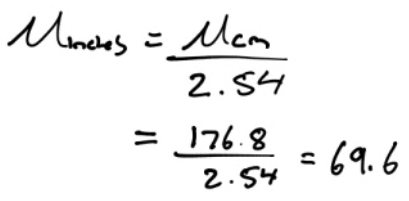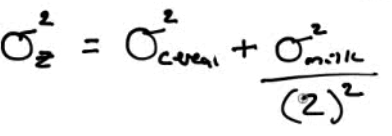# Mean and SD of Two Random Variables

• Mean• Variance# Transforming Random Variables (Linear Transformations)

• Mean• Variance# Practice Questions

• You have a sample of male/female couples. The mean height of all the woman is 65 inches, with a standard deviation of 4 inches. The mean height of all the men is 70 inches, with a standard deviation of 6 inches.

• What is the average combined height of the couples? What is the standard deviation of the combined height?• What is the average difference in the height of the couples? What is the standard deviation of the difference• A report of the National Center for Health Statistics says that the height of 20-year-old men have mean 176.8 cm and standard deviation 7.2 cm. There are 2.54 cm in an inch. What are the mean and standard deviation in inches?• The number of calories in a one-ounce serving of a breakfast cereal is a random variable with mean 110. The number of calories in a full cup of whole milk is a random variable with mean 140. For breakfast you eat one ounce of the cereal with 1/2 cup of whole milk. Let Z be the random variable that represents the total number of calories in this breakfast. What is the mean and SD of Z?• Your school has the best men's swimming team in the region. The 400-meter freestyle relay team is undefeated this year. In the 400-meter relay, each swimmer swims 100 meters. The times, in seconds, for the four swimmers this season are approximately Normally distributed with means and standard deviations as shown

Mean SD
John 55.2 2.8
Jerry 58 3
Jim 56.3 2.6
Joe 54.7 2.7
• Find the mean and standard deviation for the total team time in the 400-meter freestyle relay.

• Mean = 55.2+58+56.3+54.7 = 224.2

• Variance = 2.8^2+3^2+2.6^2+2.7^2 = 30.89

• SD = 5.6

• Find the mean and standard deviation for the average time of a single swimmer

• Mean = 224.2/4 = 56.05

• Variance = 30.89/4^2 = 1.9306

• SD = 1.39